﻿ 加热梯度泡沫材料圆板的过屈曲 Post-Buckling of Heated Gradient Foam Material Circular Plates

International Journal of Mechanics Research
Vol. 08  No. 03 ( 2019 ), Article ID: 32383 , 9 pages
10.12677/IJM.2019.83024

Post-Buckling of Heated Gradient Foam Material Circular Plates

Qinglu Li, Xin Yan

Department of Engineering Mechanics, Lanzhou University of Technology, Lanzhou Gansu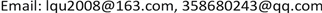Received: Sep. 6th, 2019; accepted: Sep. 20th, 2019; published: Sep. 27th, 2019ABSTRACT

The thermal post buckling behavior of graded foamed circular plates was studied. Based on the von’Kaman classical plate theory, assuming that the density of the foam gradient changes continuously along the thickness direction according to the power function, the governing equation for the buckling of a heated gradient foam plate was established. The post-buckling numerical solution of a heated foamed circular plate was solved by shooting method, and the thermal post-buckling equilibrium path and equilibrium configuration of the circular plate were given. The numerical results show that the gradient index and boundary conditions of materials have important effects on the post-buckling behavior of circular plates.1. 引言

2. 基本方程

2.1. FGMF的物性参数

$\rho \left(z\right)={\rho }_{0}\left[n{s}^{2}-\frac{n}{12}+\frac{1}{2}\right]$$n\in \left[-3,3\right]$ (1)Figure 1. Coordinate system and geometric of gradient foam circular plates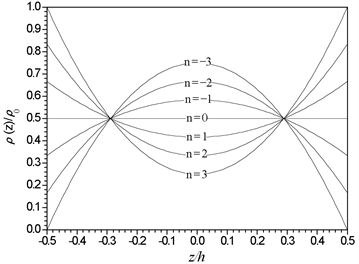Figure 2. Variations of the density along thickness of the FGMF plate for different values of n

$P\left(z\right)={P}_{0}\lambda {\left(\frac{\rho \left(z\right)}{{\rho }_{0}}\right)}^{m}$ (2)

$E\left(z\right)={E}_{0}{\left[n{\left(z/h\right)}^{2}-n/12+1/2\right]}^{2}$ (3)

$\alpha \left(z\right)={\alpha }_{0}{\left[n{\left(z/h\right)}^{2}-n/12+1/2\right]}^{2}$ (4)

2.2. 控制微分方程

${N}_{r}-{N}_{\theta }+r\frac{d{N}_{r}}{dr}=0$ (5)

$\frac{{d}^{2}{M}_{r}}{d{r}^{2}}+\frac{2}{r}\frac{d{M}_{r}}{dr}-\frac{1}{r}\frac{d{M}_{\theta }}{dr}+\frac{1}{r}\frac{d}{dr}r{N}_{r}\frac{\text{d}w}{\text{d}r}=0$ (6)

$\left({N}_{r},{N}_{\theta }\right)={\int }_{-h/2}^{h/2}\left({\sigma }_{r},{\sigma }_{\theta }\right)\text{d}z$ (7)

$\left({M}_{r},{M}_{\theta }\right)={\int }_{-h/2}^{h/2}\left({\sigma }_{r},{\sigma }_{\theta }\right)z\text{d}z$ (8)

${\epsilon }_{r}^{0}=\frac{du}{dr}+\frac{1}{2}{\left(\frac{dw}{dr}\right)}^{2}$${\epsilon }_{\theta }^{0}=\frac{u}{r}$ (9)

${\kappa }_{r}=-\frac{{d}^{2}w}{d{r}^{2}}$${\kappa }_{\theta }=-\frac{1}{r}\frac{dw}{dr}$ (10)

$\left(\begin{array}{c}{\sigma }_{r}\\ {\sigma }_{\theta }\end{array}\right)=\frac{E\left(z\right)}{1-{\nu }^{2}}\left[\begin{array}{cc}1& \nu \\ \nu & 1\end{array}\right]\left(\begin{array}{c}{\epsilon }_{r}^{0}\\ {\epsilon }_{\theta }^{0}\end{array}\right)+\frac{E\left(z\right)z}{1-{\nu }^{2}}\left[\begin{array}{cc}1& \nu \\ \nu & 1\end{array}\right]\left(\begin{array}{c}{\kappa }_{r}\\ {\kappa }_{\theta }\end{array}\right)$ (11)

$\left(\begin{array}{c}{N}_{r}\\ {N}_{\theta }\end{array}\right)=A\left[\begin{array}{cc}1& \nu \\ \nu & 1\end{array}\right]\left(\begin{array}{c}{\epsilon }_{r}^{0}\\ {\epsilon }_{\theta }^{0}\end{array}\right)+B\left[\begin{array}{cc}1& \nu \\ \nu & 1\end{array}\right]\left(\begin{array}{c}{\kappa }_{r}\\ {\kappa }_{\theta }\end{array}\right)+\left(\begin{array}{c}{N}_{T}\\ {N}_{T}\end{array}\right)$ (12)

$\left(\begin{array}{c}{M}_{r}\\ {M}_{\theta }\end{array}\right)=B\left[\begin{array}{cc}1& \nu \\ \nu & 1\end{array}\right]\left(\begin{array}{c}{\epsilon }_{r}^{0}\\ {\epsilon }_{\theta }^{0}\end{array}\right)+D\left[\begin{array}{cc}1& \nu \\ \nu & 1\end{array}\right]\left(\begin{array}{c}{\kappa }_{r}\\ {\kappa }_{\theta }\end{array}\right)+\left(\begin{array}{c}{M}_{T}\\ {M}_{T}\end{array}\right)$ (13)

$\left(A,B,D\right)={\int }_{-h/2}^{h/2}\frac{E\left(z\right)}{1-{\nu }^{2}}\left(1,z,{z}^{2}\right)\text{d}z$ (14)

$\left({N}_{T},{M}_{T}\right)={\int }_{-h/2}^{h/2}\frac{E\left(z\right)\alpha \left(z\right)T}{1-\nu }\left(1,z\right)\text{d}z$ (15)

$\left(A,B,D\right)=\frac{{E}_{0}}{1-{\nu }^{2}}\left(h{\varphi }_{1},{h}^{2}{\varphi }_{2},{h}^{3}{\varphi }_{3}\right)$ (16)

$\left({N}_{T},{M}_{T}\right)=\frac{{E}_{0}{\alpha }_{0}T}{1-\nu }\left(h{\varphi }_{4}+{h}^{2}{\varphi }_{5}\right)$ (17)

$\frac{{d}^{2}U}{d{X}^{2}}+\frac{dU}{dX}-\frac{U}{{X}^{2}}+\frac{dW}{dX}\frac{{d}^{2}W}{d{X}^{2}}+\frac{1-\nu }{2X}{\left(\frac{dW}{dX}\right)}^{2}=\frac{{\varphi }_{2}}{{\varphi }_{1}}\left(\frac{{d}^{3}W}{d{X}^{3}}+\frac{{d}^{2}W}{d{X}^{2}}-\frac{1}{{X}^{2}}\frac{dW}{dX}\right)$ (18)

$\begin{array}{l}\frac{{d}^{4}W}{d{X}^{4}}+\frac{2}{X}\frac{{d}^{3}W}{d{X}^{3}}-\frac{1}{{X}^{2}}\frac{{d}^{2}W}{d{X}^{2}}+\frac{1}{{X}^{3}}\frac{dW}{dX}\\ =\frac{{\varphi }_{1}^{2}}{{\varphi }_{1}{\varphi }_{3}-{\varphi }_{2}^{2}}\left\{\left[\frac{\nu }{X}\frac{dU}{dX}+\frac{\nu }{2X}{\left(\frac{dW}{dX}\right)}^{2}+\frac{U}{{X}^{2}}\right]\frac{dW}{dX}+\left[\frac{dU}{dX}+\frac{1}{2}{\left(\frac{dW}{dX}\right)}^{2}+\frac{\nu }{X}U\right]\frac{{d}^{2}W}{d{X}^{2}}\right\}\\ \text{\hspace{0.17em}}\text{\hspace{0.17em}}\text{ }-\frac{{\varphi }_{1}{\varphi }_{2}}{{\varphi }_{1}{\varphi }_{3}-{\varphi }_{2}^{2}}\left[{\left(\frac{{d}^{2}W}{d{X}^{2}}\right)}^{2}+\frac{1}{{X}^{2}}{\left(\frac{dW}{dX}\right)}^{2}+\frac{2\nu }{X}\frac{dW}{dX}\frac{{d}^{2}W}{d{X}^{2}}\right]-\frac{{\varphi }_{1}{\varphi }_{4}}{12\left({\varphi }_{1}{\varphi }_{3}-{\varphi }_{2}^{2}\right)}\left(\frac{1}{X}\frac{dW}{dX}+\frac{{d}^{2}W}{d{X}^{2}}\right)\tau \end{array}$ (19)

$X=\frac{r}{a}$$W=\frac{w}{h}$$U=\frac{uR}{{h}^{2}}$$\delta =\frac{a}{h}$$\tau =12\left(1+\nu \right){\alpha }_{0}T{\delta }^{2}$ (20)

2.3. 量纲归一化边界条件

$X=0$ 处， $U=0$$\frac{dW}{dX}=0$$\underset{X\to 0}{\mathrm{lim}}\left(\frac{{d}^{3}W}{d{X}^{3}}+\frac{1}{X}\frac{{d}^{2}W}{d{X}^{2}}\right)=0$ (21a)

$X=1$ 处， $W=0$$U=0$$\frac{dW}{dX}=0$ (21b)

$X=0$$U=0$$\frac{dW}{dX}=0$$\underset{X\to 0}{\mathrm{lim}}\left(\frac{{d}^{3}W}{d{X}^{3}}+\frac{1}{X}\frac{{d}^{2}W}{d{X}^{2}}\right)=0$ (22a)

$X=1$$W=0$$U=0$$\frac{dW}{dX}+\frac{1}{X}\frac{{d}^{2}W}{d{X}^{2}}=0$ (22b)

3. 数值结果与讨论

$\frac{\text{d}Y}{\text{d}\xi }=H\left(\xi ,Y\right),⤢⤢⤢\left(0<\xi <1\right)$ (23)

${B}_{0}Y\left(0\right)={b}_{0}$ , ${B}_{1}Y\left(1\right)={b}_{1}$ (24)

$Y={\left\{\begin{array}{ccccccc}{y}_{1}& {y}_{2}& {y}_{3}& {y}_{4}& {y}_{5}& {y}_{6}& {y}_{7}\end{array}\right\}}^{\text{T}}={\left\{\begin{array}{ccccccc}W& \frac{dW}{dX}& \frac{{d}^{2}W}{d{X}^{2}}& \frac{{d}^{3}W}{d{X}^{3}}& U& \frac{dU}{dX}& \lambda \end{array}\right\}}^{\text{T}}$

$\frac{dY}{dX}=H=\left({y}_{2},{y}_{3},{y}_{4},{R}_{1},{y}_{6},{R}_{2},0\right)$

${R}_{1}=-\left[\frac{{y}_{6}}{X}-\frac{{y}_{5}}{{X}^{2}}+{y}_{2}{y}_{3}+\frac{1-\nu }{2X}\right]+\frac{{\varphi }_{2}}{{\varphi }_{3}}\left[{y}_{4}+\frac{{y}_{3}}{X}-\frac{{y}_{2}}{{X}^{2}}\right]+{y}_{7}\frac{{\varphi }_{3}}{{\varphi }_{1}}$

$\begin{array}{c}{R}_{2}=-\left[\frac{2{y}_{4}}{X}-\frac{{y}_{3}}{{X}^{2}}+\frac{{y}_{2}}{{X}^{3}}\right]+\frac{{\varphi }_{1}^{2}}{{\varphi }_{1}{\varphi }_{3}-{\varphi }_{2}^{2}}\left[\frac{\nu }{X}{y}_{2}{y}_{3}+\frac{\nu }{2X}{y}_{2}^{2}+\frac{1}{{X}^{2}}{y}_{2}{y}_{5}+{y}_{3}{y}_{6}+\frac{1}{2}{y}_{2}^{2}{y}_{3}+\frac{\nu }{X}{y}_{3}{y}_{5}\right]\\ \text{\hspace{0.17em}}\text{\hspace{0.17em}}-\frac{{\varphi }_{1}{\varphi }_{2}}{{\varphi }_{1}{\varphi }_{3}-{\varphi }_{2}^{2}}\left[{y}_{3}^{2}+\frac{{y}_{2}^{2}}{{X}^{2}}+{y}_{6}+\frac{2\nu }{X}{y}_{2}{y}_{3}\right]+\frac{{\varphi }_{2}{\varphi }_{3}}{{\varphi }_{1}{\varphi }_{3}-{\varphi }_{2}^{2}}\frac{{y}_{7}}{X}\end{array}$

${B}_{0}=\left[\begin{array}{ccccccc}1& 0& 0& 0& 0& 0& 0\\ 0& 1& 0& 0& 0& 0& 0\\ 0& 0& -1/\Delta X& 0& 0& 0& 0\\ 0& 0& 0& 0& 1& 0& 0\end{array}\right]$${b}_{0}=\left\{\begin{array}{l}\xi \\ 0\\ 0\\ 0\end{array}\right\}$(25)

${B}_{1}=\left[\begin{array}{ccccccc}1& 0& 0& 0& 0& 0& 0\\ 0& 1& 0& 0& 0& 0& 0\\ 0& 0& 0& 0& 1& 0& 0\end{array}\right]$${b}_{1}=\left\{\begin{array}{l}0\\ 0\\ 0\end{array}\right\}$ (26)

${B}_{1}=\left[\begin{array}{ccccccc}1& 0& 0& 0& 0& 0& 0\\ 0& 1& 1/X& 0& 0& 0& 0\\ 0& 0& 1& 0& 0& 0& 0\end{array}\right]$${b}_{1}=\left\{\begin{array}{l}0\\ 0\\ 0\end{array}\right\}$ (27)

$\frac{\text{dZ}}{\text{d}\xi }=H\left(\xi ,Y\right)$ (28a)

$\text{Z}\left(0\right)={\left\{0,{d}_{1},0,\beta ,{d}_{2},0,{d}_{3}\right\}}^{\text{T}}=I\left(\beta ,D\right)$ (28b)

$\text{Z}\left(\xi ,\beta ,D\right)=\text{Z}\left(0\right)+{\int }_{0}^{\xi }H\left(\lambda ,\text{Z}\right)\text{d}\lambda$ (29)Table 1. Critical temperature of foam circular plates under different n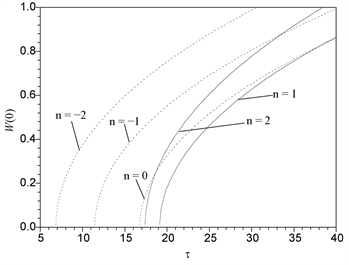Figure 3. Curves of thermal load vs. center deflection of the simply supported plate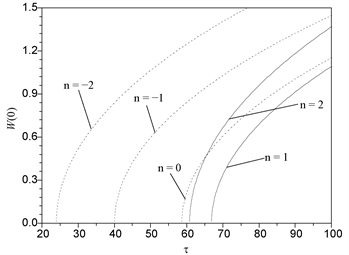Figure 4. Curves of thermal load vs. center deflection of the clamped plate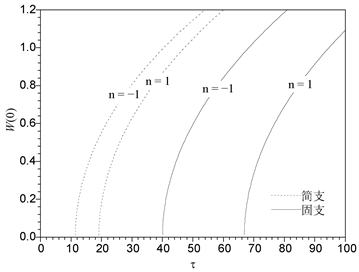Figure 5. Comparison of post-buckling equilibrium path curves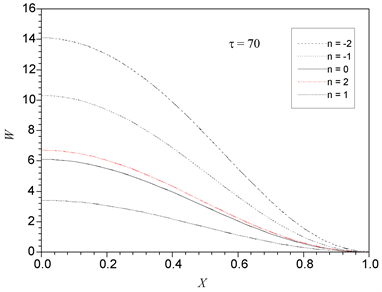Figure 6. Configuration of clamped foam plate for different values of n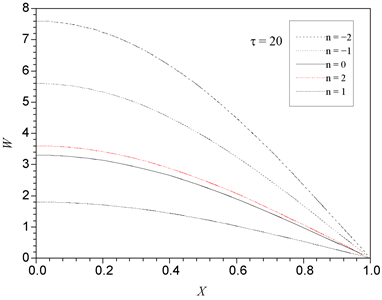Figure 7. Configuration of simply supported poam plate for different values of n

4. 结论

1) 泡沫梯度指数为负值时，随着梯度指数的增加，板的临界载荷增加；梯度指数为正值时，随梯度指数增加，临界载荷减小。梯度指数负值时的临界载荷小于梯度指数为零时的临界载荷；梯度指数正值时的临界载荷大于梯度指数为零时的临界载荷。

2) 过屈曲后，随热载荷的增加，梯度泡沫板的过屈曲行为与各向同性均匀板有很大区别，说明出泡沫梯度板的力学响应与其材料的梯度的强相关性。

3) 固支板的临界载荷大于简支板的，两种边界下板的过屈曲构形图也不相同，可见，边界条件对梯度泡沫板的稳定性也有显著影响。

Post-Buckling of Heated Gradient Foam Material Circular Plates[J]. 力学研究, 2019, 08(03): 213-221. https://doi.org/10.12677/IJM.2019.83024

1. 1. Banhartm, J. (2001) Manufacture, Characterisation and Application of Cellular Metals and Metal Foams. Progress in Materials Science, 46, 559-632.
https://doi.org/10.1016/S0079-6425(00)00002-5

2. 2. Gibson, L.J. and Ashby, M.F. (1997) Cellular Solids: Structures and Properties. Pergamon Press, Oxford.

3. 3. 卢子兴, 郭宇. 金属泡沫材料力学行为的研究概述[J]. 北京航空航天大学学报, 2003, 29(11): 978-983.

4. 4. 陈祥, 李言祥. 金属泡沫材料研究进展[J]. 材料导报, 2003, 17(5): 5-8, 11.

5. 5. 杨旭东, 石建, 程洁, 等. 填加造孔剂法制备泡沫铝及其吸能性能[J]. 航空材料学报, 2017, 37(2): 55-62.

6. 6. 卢子兴, 石上路. 低密度开孔泡沫材料力学模型的理论研究进展[J]. 力学与实践, 2015, 27(5): 13-20.

7. 7. 张健, 赵桂平, 卢天健. 泡沫金属在冲击载荷下的动态压缩行为[J]. 爆炸与冲击, 2014, 34(3): 278-284.

8. 8. 卢富德, 高德. 功能梯度泡沫动力学响应分析[J]. 振动与冲击, 2014, 33(15): 54-57.

9. 9. 关永军, 陈柳, 王金三. 材料基因组技术内涵与发展趋势[J]. 航空材料学报, 2016, 36(3): 71-78.

10. 10. 赵云松, 郭媛媛, 赵敬轩. 微量Hf对大角度晶界含Re双晶合金高温持久性能的影响[J]. 材料工程, 2019, 47(2): 76-83.

11. 11. 吕振华, 孙靖譞. 轴向变密度铝泡沫件的动态和静态压缩实验与有限元模拟分析[J]. 清华大学学报: 自然科学版, 2017, 57(7): 753-762.

12. 12. 闫长海, 孟松鹤, 陈贵清, 等. 开孔金属泡沫的传热分析[J]. 功能材料, 2006, 37(8): 1292-1302.

13. 13. 李清禄, 杨凡转, 张靖华. 热环境中非保守简支-固支FGM梁的非线性力学行为[J]. 航空材料学报, 2019, 39(1): 62-67.

14. 14. Barbier, C., Michaud, P.M., Baillis, D., et al. (2014) New Laws for the Ten-sion/Compression Properties of Voronoi Closed Cell Polymer Foams in Relation to Their Microstructure. European Journal of Mechanics—A/Solids, 45, 110-122.
https://doi.org/10.1016/j.euromechsol.2013.12.001

15. 15. Banhartm, J. (2001) Manufacture, Characterisation and Application of Cellular Metals and Metal Foams. Progress in Materials Science, 46, 559-632.
https://doi.org/10.1016/S0079-6425(00)00002-5

16. 16. Gibson, L.J. and Ashby, M.F. (1997) Cellular Solids: Structures and Properties. Pergamon Press, Oxford.

17. 17. 卢子兴, 郭宇. 金属泡沫材料力学行为的研究概述[J]. 北京航空航天大学学报, 2003, 29(11): 978-983.

18. 18. 陈祥, 李言祥. 金属泡沫材料研究进展[J]. 材料导报, 2003, 17(5): 5-8, 11.

19. 19. 杨旭东, 石建, 程洁, 等. 填加造孔剂法制备泡沫铝及其吸能性能[J]. 航空材料学报, 2017, 37(2): 55-62.

20. 20. 卢子兴, 石上路. 低密度开孔泡沫材料力学模型的理论研究进展[J]. 力学与实践, 2015, 27(5): 13-20.

21. 21. 张健, 赵桂平, 卢天健. 泡沫金属在冲击载荷下的动态压缩行为[J]. 爆炸与冲击, 2014, 34(3): 278-284.

22. 22. 卢富德, 高德. 功能梯度泡沫动力学响应分析[J]. 振动与冲击, 2014, 33(15): 54-57.

23. 23. 关永军, 陈柳, 王金三. 材料基因组技术内涵与发展趋势[J]. 航空材料学报, 2016, 36(3): 71-78.

24. 24. 赵云松, 郭媛媛, 赵敬轩. 微量Hf对大角度晶界含Re双晶合金高温持久性能的影响[J]. 材料工程, 2019, 47(2): 76-83.

25. 25. 吕振华, 孙靖譞. 轴向变密度铝泡沫件的动态和静态压缩实验与有限元模拟分析[J]. 清华大学学报: 自然科学版, 2017, 57(7): 753-762.

26. 26. 闫长海, 孟松鹤, 陈贵清, 等. 开孔金属泡沫的传热分析[J]. 功能材料, 2006, 37(8): 1292-1302.

27. 27. 李清禄, 杨凡转, 张靖华. 热环境中非保守简支-固支FGM梁的非线性力学行为[J]. 航空材料学报, 2019, 39(1): 62-67.

28. 28. Barbier, C., Michaud, P.M., Baillis, D., et al. (2014) New Laws for the Ten-sion/Compression Properties of Voronoi Closed Cell Polymer Foams in Relation to Their Microstructure. European Journal of Mechanics—A/Solids, 45, 110-122.
https://doi.org/10.1016/j.euromechsol.2013.12.001